GED Math : Numbers

Example Questions

Example Question #71 : Numbers And Operations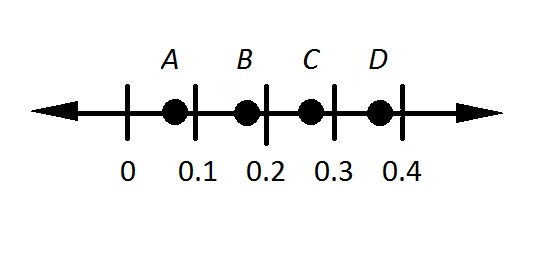Refer to the above number line. What point most likely represents the reciprocal of 2.7?

Do not use a calculator.Explanation:

2.7, expressed as a fraction, is equal to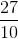. Switch the numerator and the denominator to obtain the reciprocal - this number is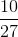. To find a decimal approximation, we divide the numerator by the denominator: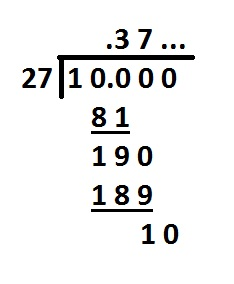The number, therefore, falls between 0.3 and 0.4, making the correct choice.

Example Question #72 : Numbers And Operations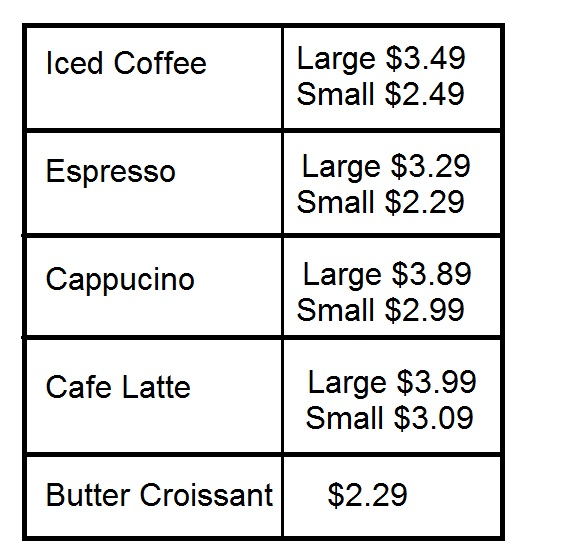Above is the menu for a coffee shop; there is no sales tax charged on purchases.

David orders a large iced coffee, two large espressos, a small cafe latte, and four butter croissants. He hands the cashier two twenty-dollar bills. How much will he get back in change?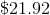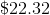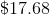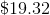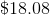Explanation:

Dave will spend $3.49 for the large iced coffee,$3.29 for each of the two large espressos, $3.09 for the cafe latte, and$2.29 for each of the butter croissants.

This is a total of: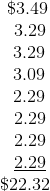Noel purchases a small drink, so he gets a $1 discount on his butter croissant. He spends$2.49 for the small iced coffee and $1.29 for the butter croissant - a total of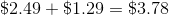. Mitch does not buy any coffee so he will not use his coupon; he will pay for the two croissants. he will spend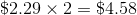. The three men, in ascending order by amount spent, are Noel, Jimi, and Mitch. Example Question #76 : Numbers And Operations Without a calculator, raise 0.4 to the third power. Possible Answers: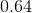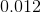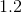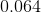Correct answer:Explanation: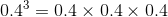First, multiply without regard to the decimal point: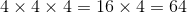Since the three factors have a total of three digits to the right of their decimal points, position the decimal point in the result so that three digits are at its right. This makes the result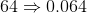. Example Question #77 : Numbers And OperationsAbove is the menu at a coffee shop. The shop is on a military base and does not charge sales tax. Zola purchases three butter croissants and three cans of cola. She hands the cashier a$20 bill and gets $10.73 back in change. How much does one can of cola cost? Possible Answers: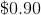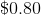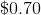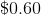Correct answer:Explanation: Zola gets$10.73 in change for a $20 bill, so the price of her food and beverages is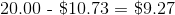. She bought three butter croissants, which cost her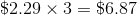. Subtract this from$9.27 to get the amount she spent on the cola.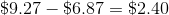She bought three cans for $2.40, so each can cost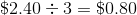. Example Question #78 : Numbers And Operations Of these four numbers, which is the greatest? Possible Answers: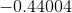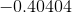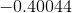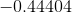Correct answer:Explanation: All four numbers are negative, so the greatest of the four will be the one with the least absolute value - that is, the one which is the least when the negative symbol is removed. Therefore, we can solve this by finding the greatest number of the set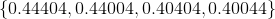. This can be done by going from left to right and examining the digits. We see that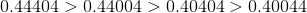, so, since the least positive number has the greatest opposite, the greatest of the original numbers is. Example Question #72 : Numbers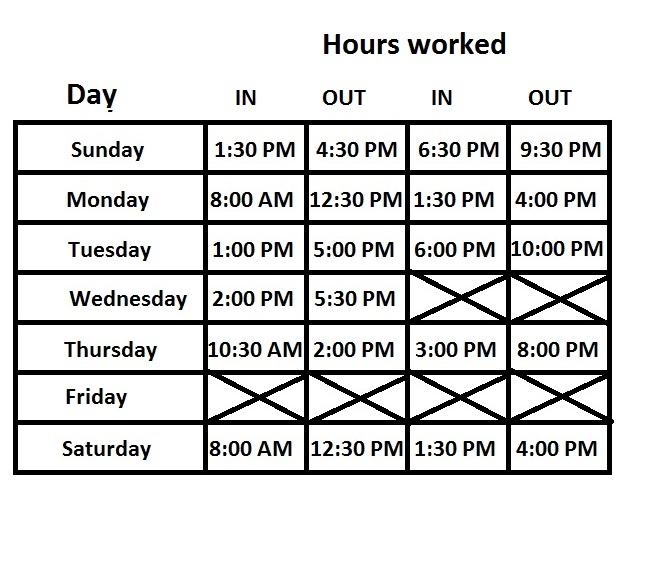Steve works for a retailer at a wage of$13.00 per hour. He normally works 40 hours per week; any hours in excess of that in any given week are paid at "time and a half" - that is, at 50% higher.

How much did Steve earn for the week reflected by the time card in the above diagram?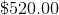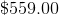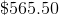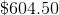Explanation:

Below is the same time card, with the number of hours worked in each shift.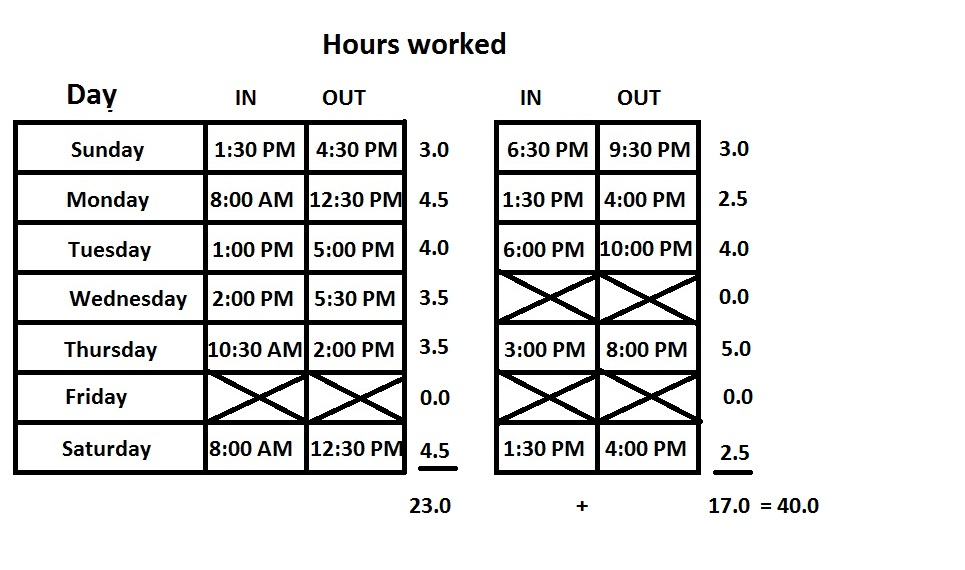Steve worked exactly 40 hours, his normal work week, so he will earn normal wage for the entire week. 40 hours at \$13.00 per hour is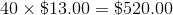.

Example Question #71 : Numbers And Operations

Each student at a classical studies school is required to take one of three languages - Latin, Greek, or Hebrew. Assume that no student takes more than one language.

One-fourth of the students decide to take Hebrew; half the remaining students decide to take Greek. What is the ratio of students who don't take Latin to those who do?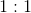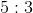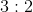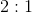Explanation:of the students take Hebrew, so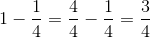of the students don't take Hebrew. Half of these students take Greek, so the other half must take Latin; this is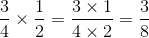of the students.

Therefore,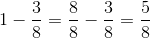of the students don't take Latin.

The ratio of those not taking Latin to those who are is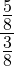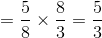.